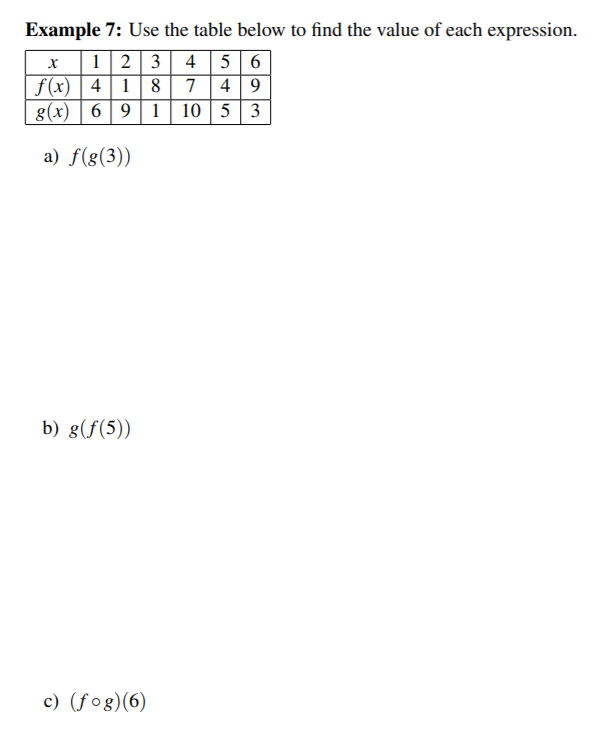# Example 7: Use the table below to find the value of each expression1 2 3 4 5 6f(x) 4 8 749g(x)6911053a) f(g(3))b) g(f(5)c) (fog)(6)

Question
1 viewshelp_outlineImage TranscriptioncloseExample 7: Use the table below to find the value of each expression 1 2 3 4 5 6 f(x) 4 8 749 g(x)6911053 a) f(g(3)) b) g(f(5) c) (fog)(6) fullscreen
check_circle

Step 1

(a) f(g(3))

We first have to find g(3) from table. For x=3 , g(3)=1

f(g(3))=f(1)

For x=1, f(1)=4

Step 2

(b) g(f(5))

We first have to find f(5) from table. For x=5 , f(5)=4

g...

### Want to see the full answer?

See Solution

#### Want to see this answer and more?

Solutions are written by subject experts who are available 24/7. Questions are typically answered within 1 hour.*

See Solution
*Response times may vary by subject and question.
Tagged in

### Other